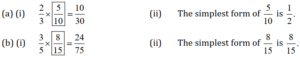# Free Ncert Solution for Class 7 Maths Chapter 2 Fractions and Decimals Exercise 2.2

Fractions and Decimals Class 7 Ex. 2.2;

New Ncert Solution for Class 7 Maths Chapter 2 Fractions and Decimals Free Solution;

Exercise 2.2

Question 1. Find :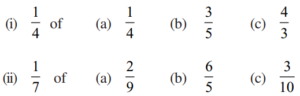Solution 1 :-

(i)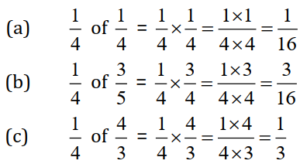(ii)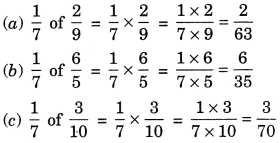Question 2 :- Multiply, and reduce to lowest-form; (if possible):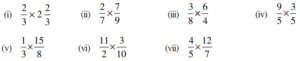Solution 2 :-

Question 3 :- Multiply the following fractions: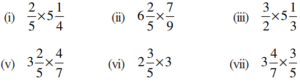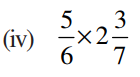Solution 3 :-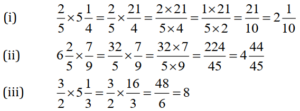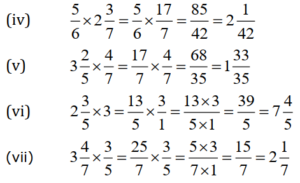Question 4 :- Which is greater: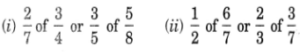Solution 4 :- (i)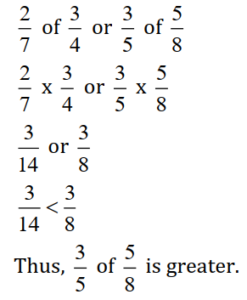(ii)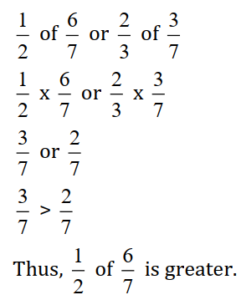Question 5 :- Saili plants, 4-saplings, in a row, in her garden. The distance between two adjacent saplings is 3/4 m. Find the distance, between the first and the last sapling.

Solution 5:-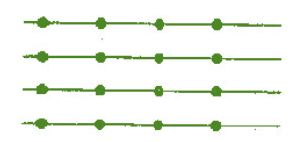Number of saplings in a row = 4
Distance between two adjacent saplings = 3/4 m
Distance between the first and the last sapling = 3 x (3/4) = 9/4 = 2(1/4) m.

Question 6 :- Lipika, reads a book for 1(3/4)-hours everyday. She reads the entire-book, in 6 days. How many hours in all were required by her, to read the book?

Solution 6 :-
In 1 day, Lipika reads = 1(3/4) hours
Since in 6 days, Lipika will need = 6×1(3/4) = 6x(7/4) = 3x(7/2) = 21/2 hours;

So, the required hours = 10(1/2) hours.

Question 7 :- A car runs, 16-km using 1-litre of petrol. How-much distance will it cover using 2(3/4) litres of petrol?

Solution 7:-
In 1 litre of petrol, the car covers distance = 16 km.
In 2(3/4) litres of petrol, the car will cover distance = 2(3/4) × 16 = (11/4)x16;

So, the required distance = 44 km.

Question 8.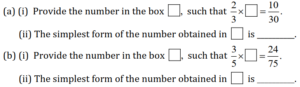Solution 8:-